# Using brms to model reaction times contaminated with errors

· 2021/04/01 · 23 minute read

Nathaniel Haines made a neat tweet showing off his model of reaction times that handles possible contamination with both implausibly short reaction times (e.g., if people make an anticipatory response that is not actually based on processing the stimulus of interest) or implausibly large reaction times (e.g., if their attention drifts away from the task, but they snap back to it after having “zoned out” for a few seconds). Response times that arise from such processes are not actually what we aim to measure in most cognitive tasks — we are instead interested in how people process and respond to a particular stimulus. Therefore, by explicitly modeling these “contamination” response times, we can get better estimates of the decision-making parameters that we actually care about. Such a model often makes more sense than just throwing away a part of the data.

Several people asked, if you can do that in brms. This started a vortex of productive procrastrination on my side - it sure should be easy to do this, right? And while Nathaniel didn’t have a brms code ready, I assure you that, yes, it is possible in brms, it is not completely straightforward, but I’ll show you the path code.

Nathaniel was kind enough to provide a bit of feedback on the post (I have no experience with reaction-time data or cogsci in general), but I should repeat that the clarity of the idea is his while all errors are mine. The overall idea of using mixtures of “real” and “contaminating” distributions to model responses is however not new - see e.g. Ratcliff & Tuerlinckx 2002.

In this model we will take a shifted lognormal representing the actual decision process and a uniform distribution modelling the contamination. For this to make sense, we need to have some upper limit on the possible contamination times, representing the maximum times we could have observed. In most cases, the limit should be larger than the maximum time we observed, although this is not strictly mathematically necessary. We then assume that each trial has a small probability of being contaminated.

Here is how generating a single data point for such a model could look in R code:

shift <- 0.1 # Shortest reaction time possible if not contaminated
mu <- log(0.5)
sigma <- 0.6
mix <- 0.06 # Probability of contamination
upper <- 5 # Maximum time of contamination

if(runif(1) < mix) {
# Contaminated
y <- runif(1, 0, upper)
} else {
# Non-contaminated
y <- shift + rlnorm(1, mu, sigma)
}

The same could be expressed in math as:

$y_i = \begin{cases} u_i & \mathrm{if} \quad z_i = 0 \\ s_i + r_i & \mathrm{if} \quad z_i = 1 \end{cases} \\ u_i \sim Uniform(0, \alpha) \\ \log(r_i) \sim Normal(\mu_i, \sigma) \\ P(z_i = 0) = \theta$

Where $$\theta$$ corresponds to mix, $$\alpha$$ to upper and $$s_i$$ to shift.

Technically, the non-contaminated signal is allowed to take values larger than upper. In practice we would however usually want upper to be large enough that larger values do not really occur.

There is one important detail in how brms does handle the shifted lognormal: brms does treat shift as unknown and estimates it, but does not allow the shift parameter to be larger than any actually observed y. We will therefore mimic this behaviour, but since we also have the contamination process, shift can in principle be larger than some y. This can potentially introduce problems for the sampler as the posterior density is not smooth when shift crosses some of the observed y values (the lognormal component is added/removed, resulting in a sharp change).

It however turns out that if shift crossing some y is rare enough, the sampling works just fine. To ensure this rarity we introduce max_shift as the upper bound for shift. In most cases, this will be the same for the whole dataset. Instead of shift, the model would then work with shiftprop = shift / max_shift - a value between 0 and 1 that is easier to work with mathematically.

Of the model parameters, we take max_shift and upper as known (but possibly differ between observations) while mu, sigma, mix and shiftprop are to be estimated and can depend on predictors. However, shiftprop is a bit complicated here and the model will make most sense if observations that have different max_shift are also allowed to have different shiftprop by putting a suitable predictor on shiftprop. Different shiftprop with the same max_shift is however definitely not an issue. So while you need to be careful with varying max_shift, varying shiftprop is OK, just note the implied logit scale. For a review on why varying shift might be important see e.g. Dully, McGovern & O’Connell 2018.

For some use cases, one could also want to set the lower bound of the contamination distribution. To keep things simple we don’t do that here, but basically the same result can then be achieved by adding/subtracting a suitable number to the response (y) and bounds (max_shift, upper)

Some experimental designs also involve a limit on the maximum time the response could have taken. In such contexts, it might make sense to treat the values as right-censored. brms supports censoring for most families, so we want our implementation to be compatible with it.

Our goal is that at the end of the post we will be able to write models like

brm(bf(y | vreal(max_shift, upper) + cens(censoring) ~ 1 + condition + (1 | subject_id),
sigma ~ condition,
mix ~ (1 | subject_id),
family = RTmixture), ...)

And let brms handle all the rest. The final result, packaged in a single file you can just load into your project is at https://github.com/martinmodrak/blog/blob/master/content/post/RTmixture.R However, be advised that the code was only quite shallowly tested, so look both ways before crossing and test if you can recover parameters from simulated data before trusting me too much.

You may know that, brms has good support for mixtures, so why not just write family = mixture(uniform, shifted_lognormal)? It turns out brms has as one of its core assumptions that every family has at least one parameter to be estimated - our uniform distribution for the contamination parameter however does not have that and thus cannot be used with brms directly. So instead we’ll have to implement a full blown custom family.

The necessary background for implementing custom families in brms can be found in the vignette on custom distributions. Here, we will explain only the more weird stuff.

## Setting up

Let’s set up and get our hands dirty.

library(cmdstanr)
library(brms)
library(tidyverse)
library(knitr)
library(patchwork)
library(bayesplot)

source("RTmixture.R")

ggplot2::theme_set(cowplot::theme_cowplot())
options(mc.cores = parallel::detectCores(), brms.backend = "cmdstanr")

cache_dir <- "_RTmixture_cache"
if(!dir.exists(cache_dir)) {
dir.create(cache_dir)
}

First, we’ll generate some fake data to test the model against. Below is just a more concise and optimized version of the random generation scheme I showed earlier.

rRTmixture <- function(n, meanlog, sdlog, mix, shift, upper) {
ifelse(runif(n) < mix,
runif(n, 0, upper),
shift + rlnorm(n, meanlog = meanlog, sdlog = sdlog))
}


Then let us generate some data

set.seed(31546522)
# Bounds of the data
max_shift <- 0.3
shift <- runif(1) * max_shift
upper <- 10
mix <- 0.1

N <- 100
Intercept <- 0.3
beta <- 0.5
X <- rnorm(N)
mu <- rep(Intercept, N) + beta * X
sigma <- 0.5

rt <- rRTmixture(N, meanlog = mu, sdlog = sigma, mix = mix, shift = shift, upper = upper)

dd <- data.frame(rt = rt, x = X, max_shift = max_shift, upper = upper)

Looking nice!

ggplot(dd, aes(x = rt)) + geom_density()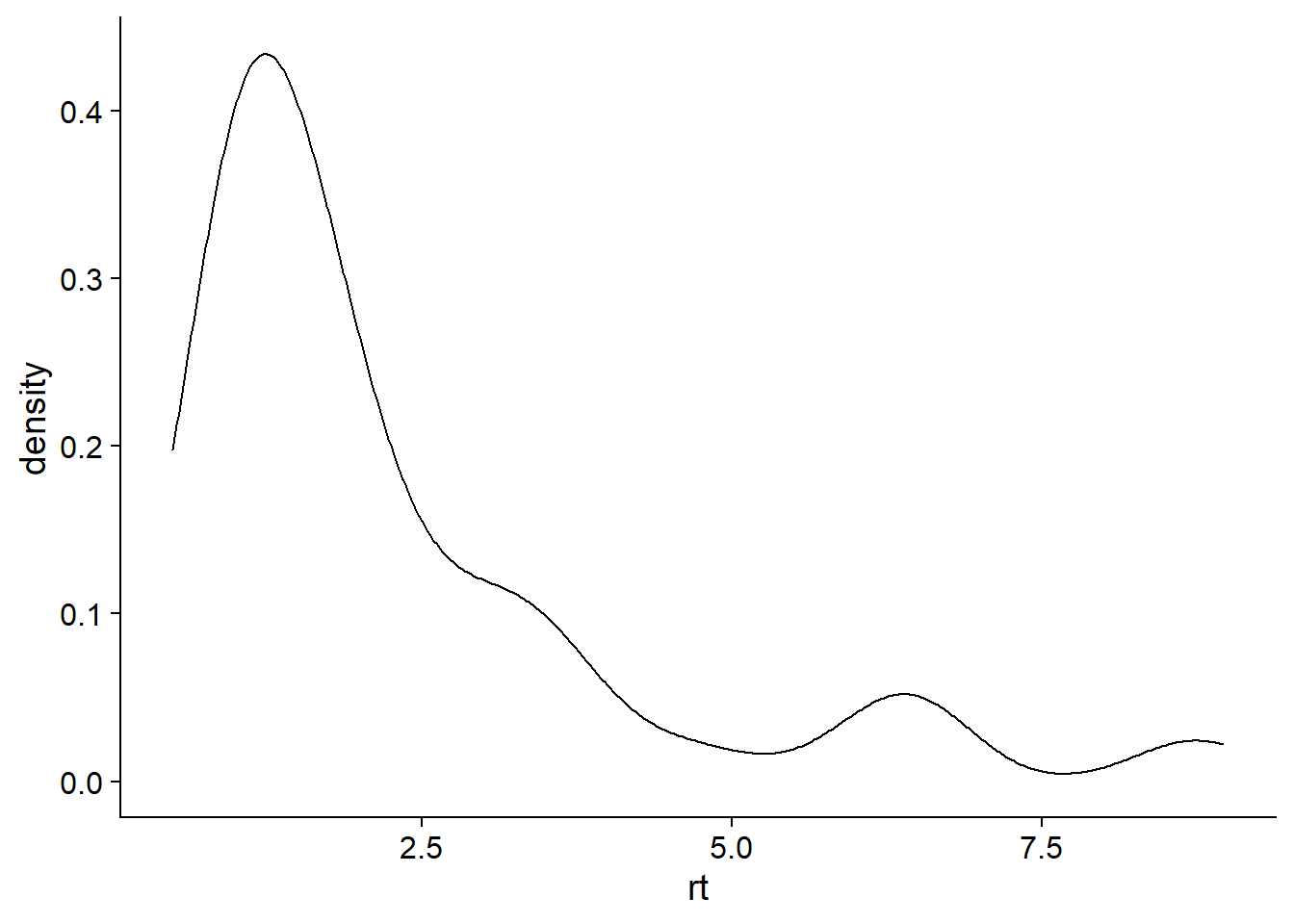## Core of the family

Now we need the Stan implementation of the family. That is probably the most technical part. Stan user’s guide has some background on mixture models in Stan. We’ll note that times before shift can only come from the uniform component and times after upper can only come from the lognormal component. For others we mix both a lognormal and the uniform via log_mix.

With the Stan code ready, we then define the parameters of the distribution in a way that brms understands.

stan_funs_base <- stanvar(block = "functions", scode = "
real RTmixture_lpdf(real y, real mu, real sigma, real mix,
real shiftprop, real max_shift, real upper) {
real shift = shiftprop * max_shift;
if(y <= shift) {
// Could only be created by the contamination
return log(mix) + uniform_lpdf(y | 0, upper);
} else if(y >= upper) {
// Could only come from the lognormal
return log1m(mix) + lognormal_lpdf(y - shift | mu, sigma);
} else {
// Actually mixing
real lognormal_llh = lognormal_lpdf(y - shift | mu, sigma);
real uniform_llh = uniform_lpdf(y | 0, upper);
return log_mix(mix, uniform_llh, lognormal_llh);
}
}

")

RTmixture <- custom_family(
"RTmixture",
dpars = c("mu", "sigma", "mix", "shiftprop"), # Those will be estimated
links = c("identity", "log", "logit", "logit"),
type = "real",
lb = c(NA, 0, 0, 0), # bounds for the parameters
ub = c(NA, NA, 1, 1),
vars = c("vreal1[n]", "vreal2[n]") # Data for max_shift and upper (known)
) 

And we are ready to fit! We will put a weakly informative beta(1,5) prior on the proportion of contamination - this means we a prior believe that there is a 95% chance that the contamination is lower than qbeta(0.95, 1, 5) = 0.4507197. One could definitely be justified in tightening this prior even further toward zero for many tasks. vreal is just brms’s way of annotating arbitrary additional data for the distribution. We need to pass both the family and the associated stanvars.

fit_mix <- brm(rt | vreal(max_shift, upper) ~ x, data = dd, family = RTmixture,
stanvars = stan_funs_base,
refresh = 0,
file = paste0(cache_dir, "/mix"), file_refit = "on_change",
prior = c(prior(beta(1, 5), class = "mix")))
fit_mix
##  Family: RTmixture
##   Links: mu = identity; sigma = identity; mix = identity; shiftprop = identity
## Formula: rt | vreal(max_shift, upper) ~ x
##    Data: dd (Number of observations: 100)
##   Draws: 4 chains, each with iter = 1000; warmup = 0; thin = 1;
##          total post-warmup draws = 4000
##
## Population-Level Effects:
##           Estimate Est.Error l-95% CI u-95% CI Rhat Bulk_ESS Tail_ESS
## Intercept     0.33      0.07     0.20     0.48 1.00     2097     2242
## x             0.44      0.05     0.33     0.54 1.00     2368     2460
##
## Family Specific Parameters:
##           Estimate Est.Error l-95% CI u-95% CI Rhat Bulk_ESS Tail_ESS
## sigma         0.45      0.05     0.36     0.56 1.00     2379     1976
## mix           0.13      0.05     0.05     0.24 1.00     3159     2589
## shiftprop     0.82      0.18     0.32     1.00 1.00     1640     1623
##
## Draws were sampled using sample(hmc). For each parameter, Bulk_ESS
## and Tail_ESS are effective sample size measures, and Rhat is the potential
## scale reduction factor on split chains (at convergence, Rhat = 1).

We note that we have quite good recovery of the effect of x (simulated as 0.5) and of sigma (which was 0.5), but 100 observations are not enough to constrain the mix parameter really well (simulated as 0.1).

For comparison, we also fit the default shifted lognormal as implemented in brms.

fit_base <- brm(rt ~ x, data = dd, family = shifted_lognormal, refresh = 0,
file = paste0(cache_dir, "/base"), file_refit = "on_change")

fit_base
##  Family: shifted_lognormal
##   Links: mu = identity; sigma = identity; ndt = identity
## Formula: rt ~ x
##    Data: dd (Number of observations: 100)
##   Draws: 4 chains, each with iter = 1000; warmup = 0; thin = 1;
##          total post-warmup draws = 4000
##
## Population-Level Effects:
##           Estimate Est.Error l-95% CI u-95% CI Rhat Bulk_ESS Tail_ESS
## Intercept     0.34      0.09     0.16     0.53 1.00     1958     1949
## x             0.47      0.07     0.33     0.61 1.00     2711     2495
##
## Family Specific Parameters:
##       Estimate Est.Error l-95% CI u-95% CI Rhat Bulk_ESS Tail_ESS
## sigma     0.69      0.07     0.56     0.82 1.00     1883     1802
## ndt       0.34      0.07     0.17     0.44 1.00     1634     1420
##
## Draws were sampled using sample(hmc). For each parameter, Bulk_ESS
## and Tail_ESS are effective sample size measures, and Rhat is the potential
## scale reduction factor on split chains (at convergence, Rhat = 1).

We see that the inferences for sigma are a bit biased but this is not necessarily only due to the mixture, another potentially biasing is the different handling of the shift.

## Censoring + constant shift

To support censoring in brms the family has to come with log CDF (cumulative distribution function) and log CCDF (complementary CDF) implementations in Stan, which we provide below. Those match the _lpdf pretty closely.


stan_funs <- stan_funs_base + stanvar(block = "functions", scode = "
real RTmixture_lcdf(real y, real mu, real sigma, real mix,
real shiftprop, real max_shift, real upper) {
real shift = shiftprop * max_shift;
if(y <= shift) {
return log(mix) + uniform_lcdf(y | 0, upper);
} else if(y >= upper) {
// The whole uniform part is below, so the mixture part is log(1) = 0
return log_mix(mix, 0, lognormal_lcdf(y - shift | mu, sigma));
} else {
real lognormal_llh = lognormal_lcdf(y - shift | mu, sigma);
real uniform_llh = uniform_lcdf(y | 0, upper);
return log_mix(mix, uniform_llh, lognormal_llh);
}
}

real RTmixture_lccdf(real y, real mu, real sigma, real mix,
real shiftprop, real max_shift, real upper) {

real shift = shiftprop * max_shift;
if(y <= shift) {
// The whole lognormal part is above, so the mixture part is log(1) = 0
return log_mix(mix, uniform_lccdf(y | 0, upper), 0);
} else if(y >= upper) {
return log1m(mix) + lognormal_lccdf(y - shift | mu, sigma);
} else {
real lognormal_llh = lognormal_lccdf(y - shift | mu, sigma);
real uniform_llh = uniform_lccdf(y | 0, upper);
return log_mix(mix, uniform_llh, lognormal_llh);
}

}
")


To test if this work, we’ll do quite aggressive censoring and treat anything larger than 1.5 as censored. In most cases it makes sense to have upper be the same as the censoring bound, so we’ll do that

set.seed(25462255)
shift <- 0.15
cens_bound <- upper <- 1.5
mix <- 0.08

N <- 110
Intercept <- 0.5
beta <- -0.3
X <- rnorm(N)
mu <- rep(Intercept, N) + beta * X
sigma <- 0.4

rt <- rRTmixture(N, meanlog = mu, sdlog = sigma,
mix = mix, shift = shift, upper = upper)
censored <- rt > cens_bound
rt[censored] <- cens_bound

dd_cens <- data.frame(rt = rt,
censored = if_else(censored, "right", "none"),
x = X, max_shift = shift, upper = upper)

Finally, this model starts to be problematic if we try to estimate shift (well, actually shiftprop) as well. An easy way to to make shift always equal to max_shift is to set a constant prior on shiftprop, as we do below.

fit_mix_cens <- brm(rt | vreal(max_shift, upper) + cens(censored) ~ x,
data = dd_cens,
family = RTmixture,
stanvars = stan_funs,
refresh = 0,
file = paste0(cache_dir, "/mix_cens"),
file_refit = "on_change",
prior = c(prior(beta(1, 5), class = "mix"),
prior(constant(1), class = "shiftprop")))
fit_mix_cens
##  Family: RTmixture
##   Links: mu = identity; sigma = identity; mix = identity; shiftprop = identity
## Formula: rt | vreal(max_shift, upper) + cens(censored) ~ x
##    Data: dd_cens (Number of observations: 110)
##   Draws: 4 chains, each with iter = 1000; warmup = 0; thin = 1;
##          total post-warmup draws = 4000
##
## Population-Level Effects:
##           Estimate Est.Error l-95% CI u-95% CI Rhat Bulk_ESS Tail_ESS
## Intercept     0.46      0.08     0.34     0.65 1.00     2022     1674
## x            -0.23      0.07    -0.38    -0.12 1.00     2094     1606
##
## Family Specific Parameters:
##           Estimate Est.Error l-95% CI u-95% CI Rhat Bulk_ESS Tail_ESS
## sigma         0.36      0.07     0.26     0.53 1.00     2019     2565
## mix           0.15      0.05     0.07     0.25 1.00     2943     2595
## shiftprop     1.00      0.00     1.00     1.00   NA       NA       NA
##
## Draws were sampled using sample(hmc). For each parameter, Bulk_ESS
## and Tail_ESS are effective sample size measures, and Rhat is the potential
## scale reduction factor on split chains (at convergence, Rhat = 1).

It works and the inferences are reasonably close to what we simulated with. A more thorough evaluation would require simulation-based calibration, which would be nice, but would require a bit more energy than I have now. But it seems that at least the models are not completely wrong.

If you want to model varying shift but having issues fitting, it might make sense to adjust max_shift on a per-group basis to have max_shift larger only than a small proportion of observations in this group. As noted above, if you set different max_shift per for example subject_id, you should also have shiftprop ~ subject_id or the model might not make sense.

## Making predictions

We successfully fitted a few models, but there are some tweaks we need to do to make full use of the family. We might for example want to make predictions - e.g. to make posterior predictive checks - so we also need to implement prediction code. You’ll notice that we are just extracting the parameters from the prepared predictions and passing those to the generator function we defined earlier.


posterior_predict_RTmixture <- function(i, prep, ...) {
if((!is.null(prep$data$lb) && prep$data$lb[i] > 0) ||
(!is.null(prep$data$ub) && prep$data$ub[i] < Inf)) {
stop("Predictions for truncated distributions not supported")
}

mu <- brms:::get_dpar(prep, "mu", i = i)
sigma <- brms:::get_dpar(prep, "sigma", i = i)
mix <- brms:::get_dpar(prep, "mix", i = i)
shiftprop <- brms:::get_dpar(prep, "shiftprop", i = i)

max_shift <- prep$data$vreal1[i]
upper <- prep$data$vreal2[i]
shift = shiftprop * max_shift

rRTmixture(prep$ndraws, meanlog = mu, sdlog = sigma, mix = mix, shift = shift, upper = upper) }  Note that the get_dpar helper that simplifies some bookeeping is currently internal in brms, but will be exposed in upcoming release. With that, we can do a posterior predictive check for both models. We use only single core for predictions, because on Windows, multicore is slow and will not be able to access the custom prediction functions. pp_mix <- pp_check(fit_mix, type = "dens_overlay", ndraws = 100, cores = 1) + ggtitle("Mixture") pp_base <- pp_check(fit_base, type = "dens_overlay", ndraws = 100, cores = 1) + ggtitle("Shifted lognormal") pp_mix / pp_base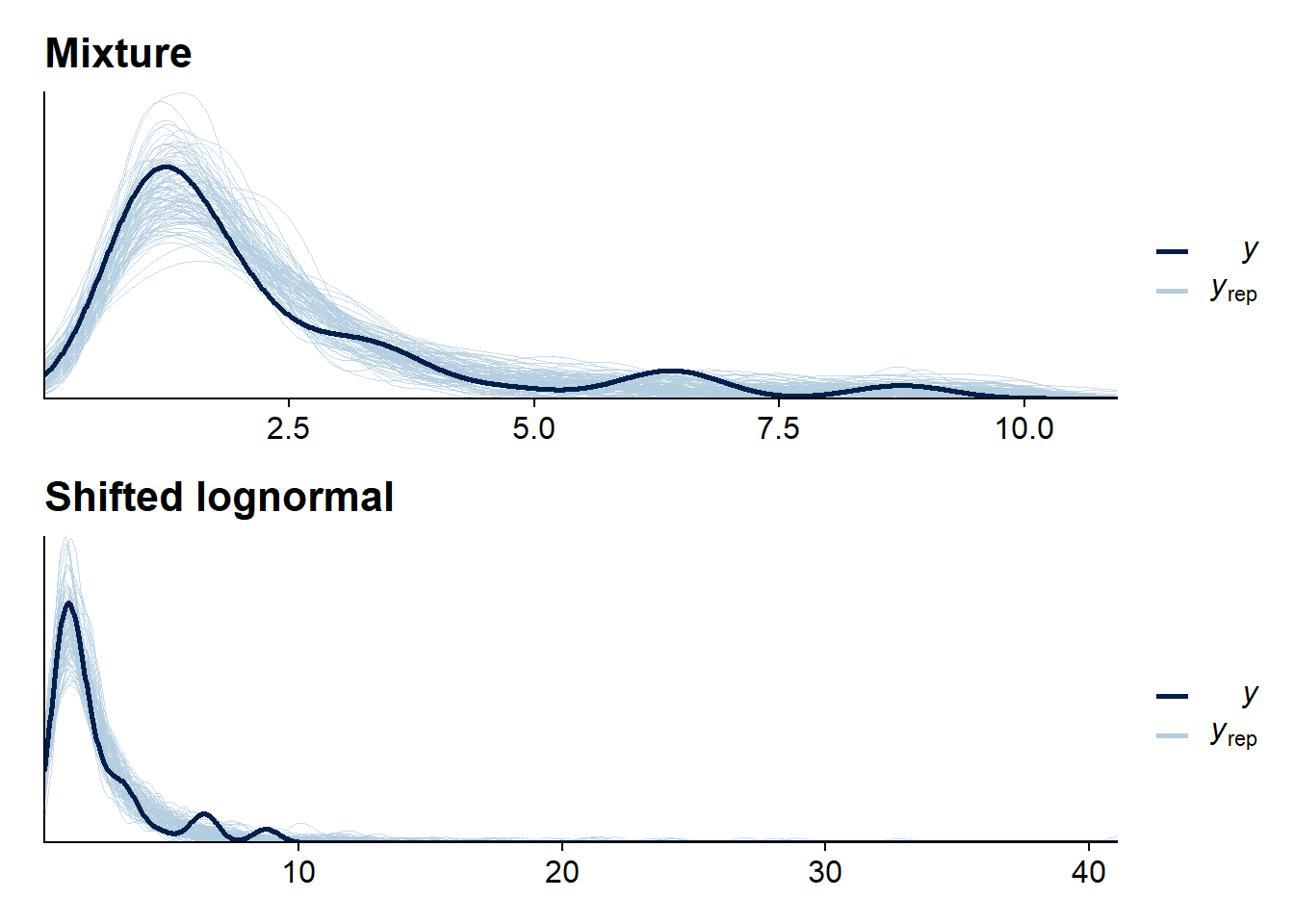For this dataset, the mixture is not doing that much in improving the bulk of the predictions, but it manages to avoid the very long tail the lognormal-only model needs to accomodate the larger values. We might also look at checks of the censored model. brms does not directly support predicting censored variables (because the data passed to the model are not enough to completely determine all censoring), but we can easily do this manually: set.seed(123566) pred_cens <- posterior_predict(fit_mix_cens, cores = 1) pred_cens_cens <- pred_cens # Do the censoring pred_cens_cens[pred_cens > cens_bound] <- cens_bound samples_dens <- sample(1:(dim(pred_cens)), size = 50) ppc_cens1 <- ppc_dens_overlay(dd_cens$rt, pred_cens_cens[samples_dens,])  +
ggtitle("Censored dataset")
ppc_cens2 <- ppc_stat(1.0 * (dd_cens$censored == "right"), 1.0 * (pred_cens >= cens_bound), binwidth = 0.02) + ggtitle("Proportion censored") ppc_cens1 + ppc_cens2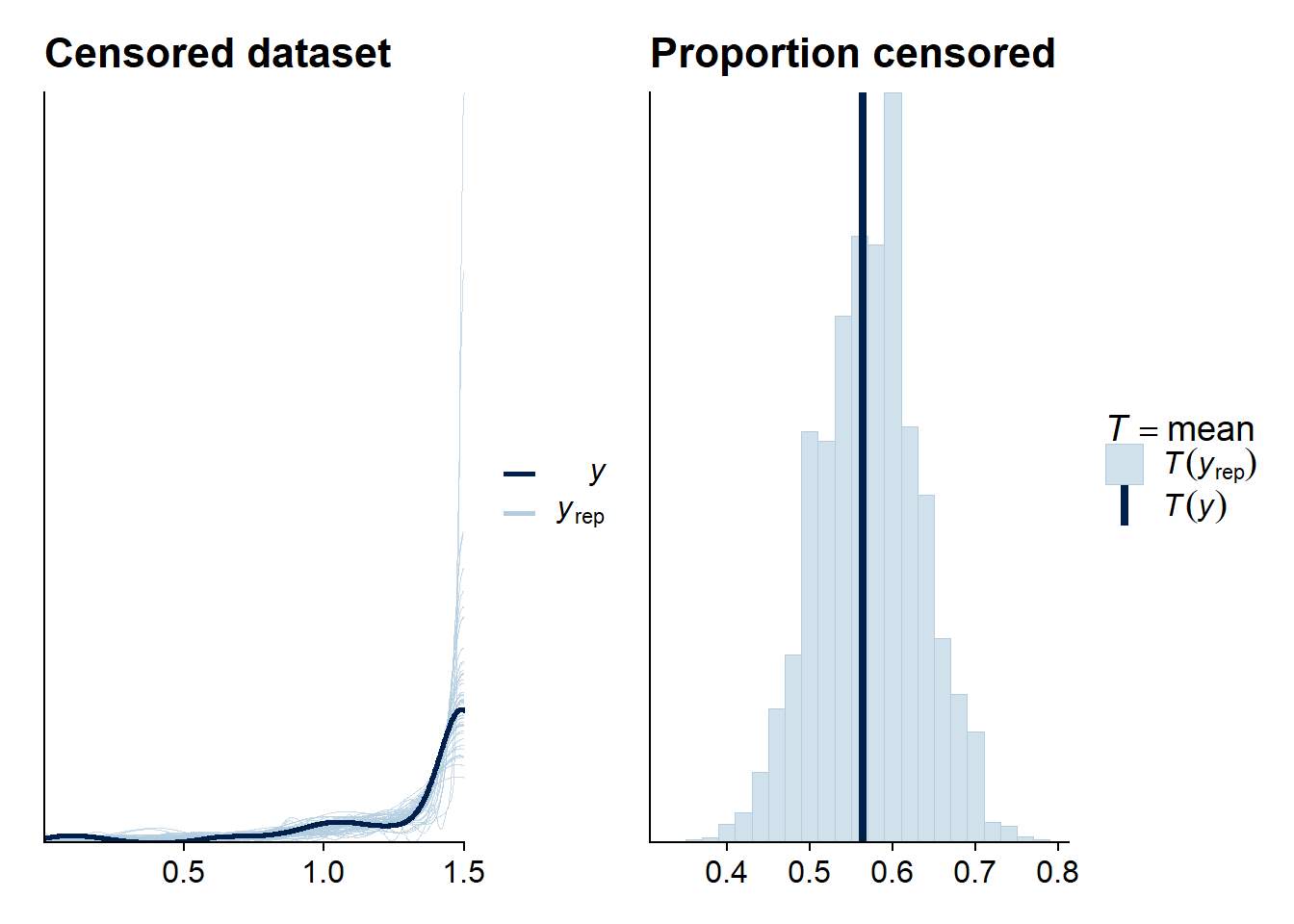The model seems to do OK. ## Using loo Similarly, we might want to do model comparison or stacking with loo, so we also implement the log_lik function. ## Needed for numerical stability ## from http://tr.im/hH5A logsumexp <- function (x) { y = max(x) y + log(sum(exp(x - y))) } RTmixture_lpdf <- function(y, meanlog, sdlog, mix, shift, upper) { unif_llh = dunif(y , min = 0, max = upper, log = TRUE) lognormal_llh = dlnorm(y - shift, meanlog = meanlog, sdlog = sdlog, log = TRUE) - plnorm(upper - shift, meanlog = meanlog, sdlog = sdlog, log.p = TRUE) # Computing logsumexp(log(mix) + unif_llh, log1p(-mix) + lognormal_llh) # but vectorized llh_matrix <- array(NA_real_, dim = c(2, max(length(unif_llh), length(lognormal_llh)))) llh_matrix[1,] <- log(mix) + unif_llh llh_matrix[2,] <- log1p(-mix) + lognormal_llh apply(llh_matrix, MARGIN = 2, FUN = logsumexp) } log_lik_RTmixture <- function(i, draws) { mu <- brms:::get_dpar(draws, "mu", i = i) sigma <- brms:::get_dpar(draws, "sigma", i = i) mix <- brms:::get_dpar(draws, "mix", i = i) shiftprop <- brms:::get_dpar(draws, "shiftprop", i = i) max_shift <- draws$data$vreal1[i] upper <- draws$data$vreal2[i] shift = shiftprop * max_shift y <- draws$data$Y[i] RTmixture_lpdf(y, meanlog = mu, sdlog = sigma, mix = mix, shift = shift, upper = upper) }  And now, we can compare the models: fit_mix <- add_criterion(fit_mix, "loo", cores = 1) fit_base <- add_criterion(fit_base, "loo", cores = 1) loo_compare(fit_mix, fit_base) ## elpd_diff se_diff ## fit_mix 0.0 0.0 ## fit_base -7.5 5.3 No surprise here - we simulated the data with the mixture model and indeed, this is preferred to a different model. Also, the shifted-lognormal model has one very influential observation, which turns out to be the smallest observed reaction time. dd$rt[fit_base$criteria$loo$diagnostics$pareto_k > 0.7]
##  0.4909057
min(dd$rt) ##  0.4909057 This once again shows that the lognormal has problem accomodating both the high and low contamination (while it is plausible it could accomodate a small amount of just high or just low contamination quite well). ## Crazy models Since brms is great, we can now do all sorts of stuff like put predictors on the mix parameter - e.g. to get a per-subject estimate of the amount of contamination. To do this, we’ll also put a weakly informative prior on the intercept for the mixture that assumes low contamination and we don’t expect huge variability in the amount of contamination (with wider priors the model starts to diverge as we would need much more data to constrain it well). set.seed(35486622) dd_subj <- dd_cens dd_subj$subject_id <- sample(1:12, size = nrow(dd_cens), replace = TRUE)

fit_mix_all <- brm(
bf(rt | vreal(max_shift, upper) + cens(censored) ~ x,
mix ~ 1 + (1 | subject_id),
family = RTmixture),
data = dd_subj,
stanvars = stan_funs,
refresh = 0,
file = paste0(cache_dir, "/mix_all"), file_refit = "on_change",
prior = c(prior(normal(-3, 1), class = "Intercept", dpar = "mix"),
prior(normal(0,0.5), class = "sd", dpar = "mix"),
prior(constant(1), class = "shiftprop")))

fit_mix_all
##  Family: RTmixture
##   Links: mu = identity; sigma = identity; mix = logit; shiftprop = identity
## Formula: rt | vreal(max_shift, upper) + cens(censored) ~ x
##          mix ~ 1 + (1 | subject_id)
##    Data: dd_subj (Number of observations: 110)
##   Draws: 4 chains, each with iter = 1000; warmup = 0; thin = 1;
##          total post-warmup draws = 4000
##
## Group-Level Effects:
## ~subject_id (Number of levels: 12)
##                   Estimate Est.Error l-95% CI u-95% CI Rhat Bulk_ESS Tail_ESS
## sd(mix_Intercept)     0.38      0.29     0.02     1.07 1.00     2706     2137
##
## Population-Level Effects:
##               Estimate Est.Error l-95% CI u-95% CI Rhat Bulk_ESS Tail_ESS
## Intercept         0.45      0.07     0.33     0.62 1.00     3586     2305
## mix_Intercept    -2.05      0.44    -2.96    -1.25 1.00     4207     2807
## x                -0.22      0.06    -0.37    -0.11 1.00     3715     2666
##
## Family Specific Parameters:
##           Estimate Est.Error l-95% CI u-95% CI Rhat Bulk_ESS Tail_ESS
## sigma         0.37      0.07     0.26     0.54 1.00     3534     3025
## shiftprop     1.00      0.00     1.00     1.00   NA       NA       NA
##
## Draws were sampled using sample(hmc). For each parameter, Bulk_ESS
## and Tail_ESS are effective sample size measures, and Rhat is the potential
## scale reduction factor on split chains (at convergence, Rhat = 1).

Checking that posterior predictions work:

set.seed(1233354)
pred_cens <- posterior_predict(fit_mix_all, cores = 1)
pred_cens_cens <- pred_cens
pred_cens_cens[pred_cens > cens_bound] <- cens_bound
samples_dens <- sample(1:(dim(pred_cens)), size = 50)
ppc_dens_overlay(dd_cens$rt, pred_cens_cens[samples_dens,])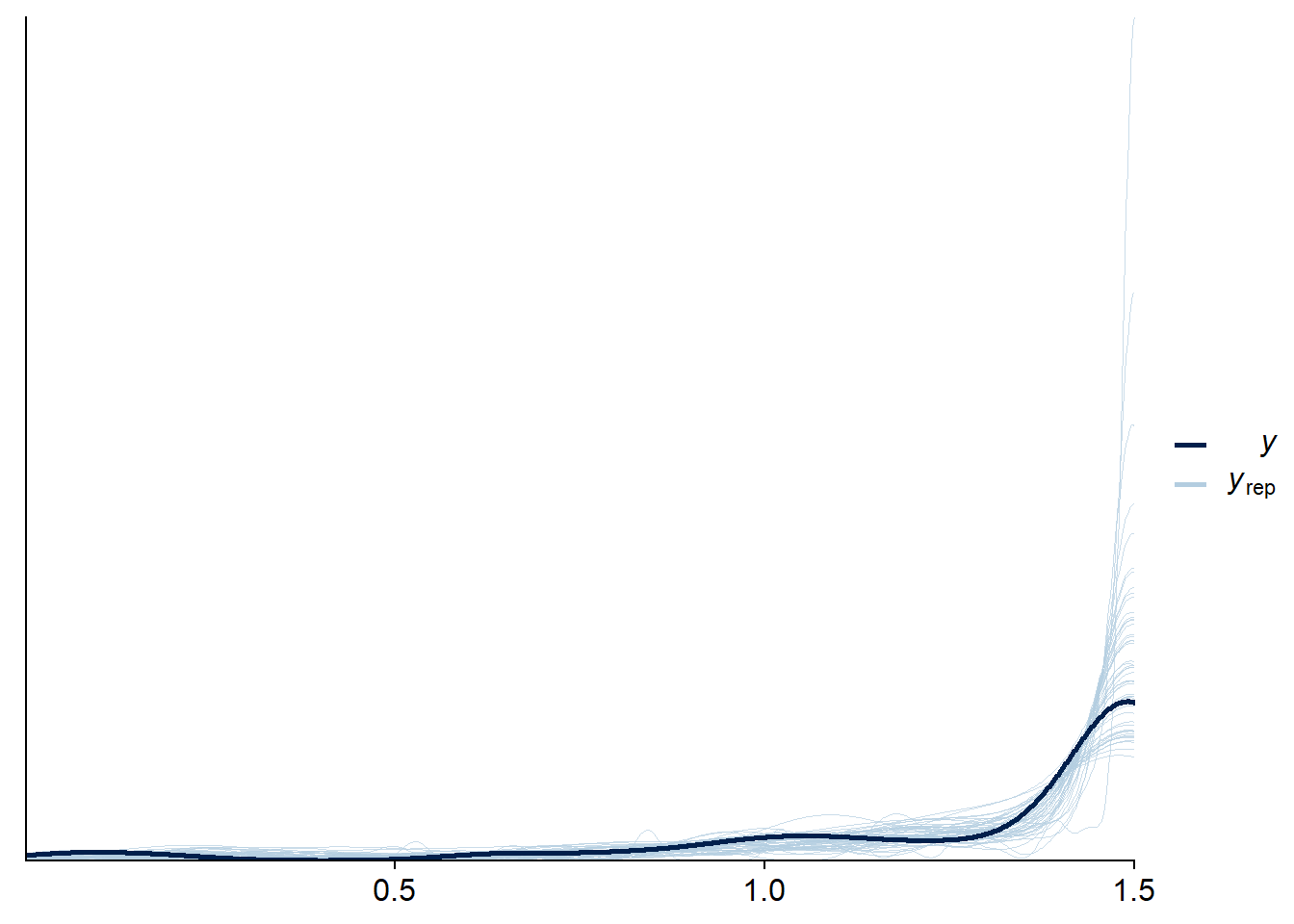We can also do multivariate models where some of the predictors are correlated across answers: set.seed(0245562) # Build a dataset containing two separate predictions dd_both <- dd dd_both$rt2 <- dd_cens$rt[1:nrow(dd_both)] dd_both$x2 <- dd_cens$x[1:nrow(dd_both)] dd_both$censored2 <- dd_cens$censored[1:nrow(dd_both)] dd_both$max_shift2 <- dd_cens$max_shift[1:nrow(dd_both)] dd_both$upper2 <- dd_cens$upper[1:nrow(dd_both)] dd_both$subject_id <- sample(1:12, size = nrow(dd_both), replace = TRUE)

fit_mix_multivar <- brm(
bf(rt | vreal(max_shift, upper)  ~ x,
mix ~ 1 + (1 | p | subject_id),
family = RTmixture) +
bf(rt2 | vreal(max_shift2, upper2) + cens(censored2) ~ x2,
mix ~ 1 + (1 | p | subject_id),
family = RTmixture),
data = dd_both,
stanvars = stan_funs,
refresh = 0,
file = paste0(cache_dir, "/mix_multivar"), file_refit = "on_change",
prior = c(prior(normal(-3, 1), class = "Intercept", dpar = "mix", resp = "rt"),
prior(normal(0,0.5), class = "sd", dpar = "mix", resp = "rt"),
prior(constant(1), class = "shiftprop", resp = "rt"),
prior(normal(-3, 1), class = "Intercept", dpar = "mix", resp = "rt2"),
prior(normal(0,0.5), class = "sd", dpar = "mix", resp = "rt2"),
prior(constant(1), class = "shiftprop", resp = "rt2")
),
)
## Setting 'rescor' to FALSE by default for this model
fit_mix_multivar
##  Family: MV(RTmixture, RTmixture)
##   Links: mu = identity; sigma = identity; mix = logit; shiftprop = identity
##          mu = identity; sigma = identity; mix = logit; shiftprop = identity
## Formula: rt | vreal(max_shift, upper) ~ x
##          mix ~ 1 + (1 | p | subject_id)
##          rt2 | vreal(max_shift2, upper2) + cens(censored2) ~ x2
##          mix ~ 1 + (1 | p | subject_id)
##    Data: dd_both (Number of observations: 100)
##   Draws: 4 chains, each with iter = 1000; warmup = 0; thin = 1;
##          total post-warmup draws = 4000
##
## Group-Level Effects:
## ~subject_id (Number of levels: 12)
##                                         Estimate Est.Error l-95% CI u-95% CI
## sd(mix_rt_Intercept)                        0.33      0.25     0.01     0.91
## sd(mix_rt2_Intercept)                       0.37      0.27     0.02     1.01
## cor(mix_rt_Intercept,mix_rt2_Intercept)     0.00      0.58    -0.95     0.95
##                                         Rhat Bulk_ESS Tail_ESS
## sd(mix_rt_Intercept)                    1.00     2713     1787
## sd(mix_rt2_Intercept)                   1.00     2587     2046
## cor(mix_rt_Intercept,mix_rt2_Intercept) 1.00     4331     2836
##
## Population-Level Effects:
##                   Estimate Est.Error l-95% CI u-95% CI Rhat Bulk_ESS Tail_ESS
## rt_Intercept          0.30      0.06     0.18     0.43 1.00     4743     3085
## mix_rt_Intercept     -2.28      0.54    -3.50    -1.35 1.00     4035     1843
## rt2_Intercept         0.43      0.08     0.31     0.60 1.00     3258     1826
## mix_rt2_Intercept    -1.91      0.44    -2.80    -1.11 1.00     4456     3016
## rt_x                  0.46      0.05     0.36     0.56 1.00     5440     2978
## rt2_x2               -0.25      0.07    -0.40    -0.13 1.00     3316     2106
##
## Family Specific Parameters:
##               Estimate Est.Error l-95% CI u-95% CI Rhat Bulk_ESS Tail_ESS
## sigma_rt          0.49      0.05     0.40     0.60 1.00     4907     3014
## sigma_rt2         0.36      0.07     0.25     0.53 1.00     3449     2885
## shiftprop_rt      1.00      0.00     1.00     1.00   NA       NA       NA
## shiftprop_rt2     1.00      0.00     1.00     1.00   NA       NA       NA
##
## Draws were sampled using sample(hmc). For each parameter, Bulk_ESS
## and Tail_ESS are effective sample size measures, and Rhat is the potential
## scale reduction factor on split chains (at convergence, Rhat = 1).

Testing that predictions work even for multivariate models. Note that we don’t bother with censoring for rt2 so the predictions look wrong.

pp_check(fit_mix_multivar, resp = "rt", ndraws = 30, cores = 1)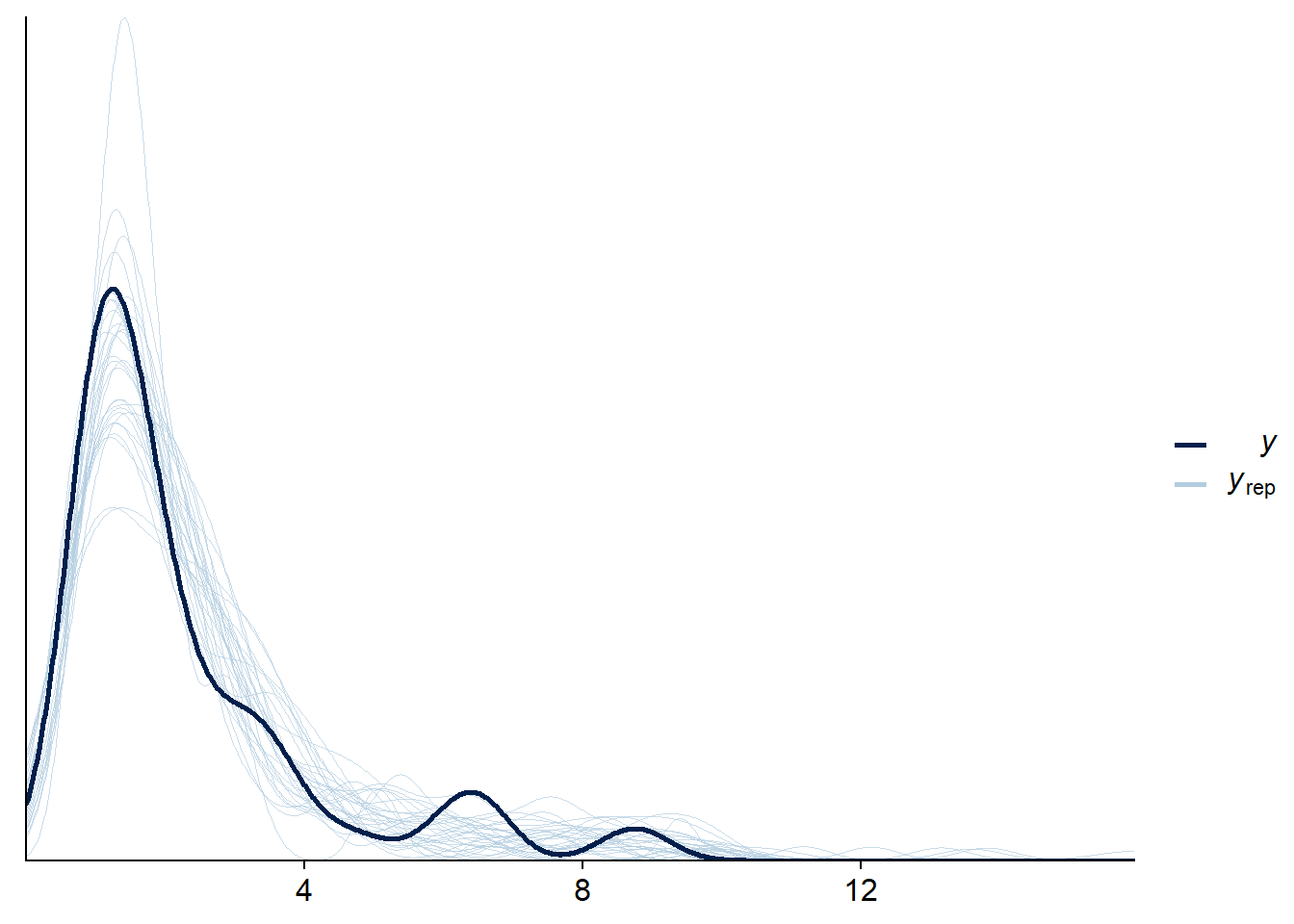pp_check(fit_mix_multivar, resp = "rt2", ndraws = 30, cores = 1)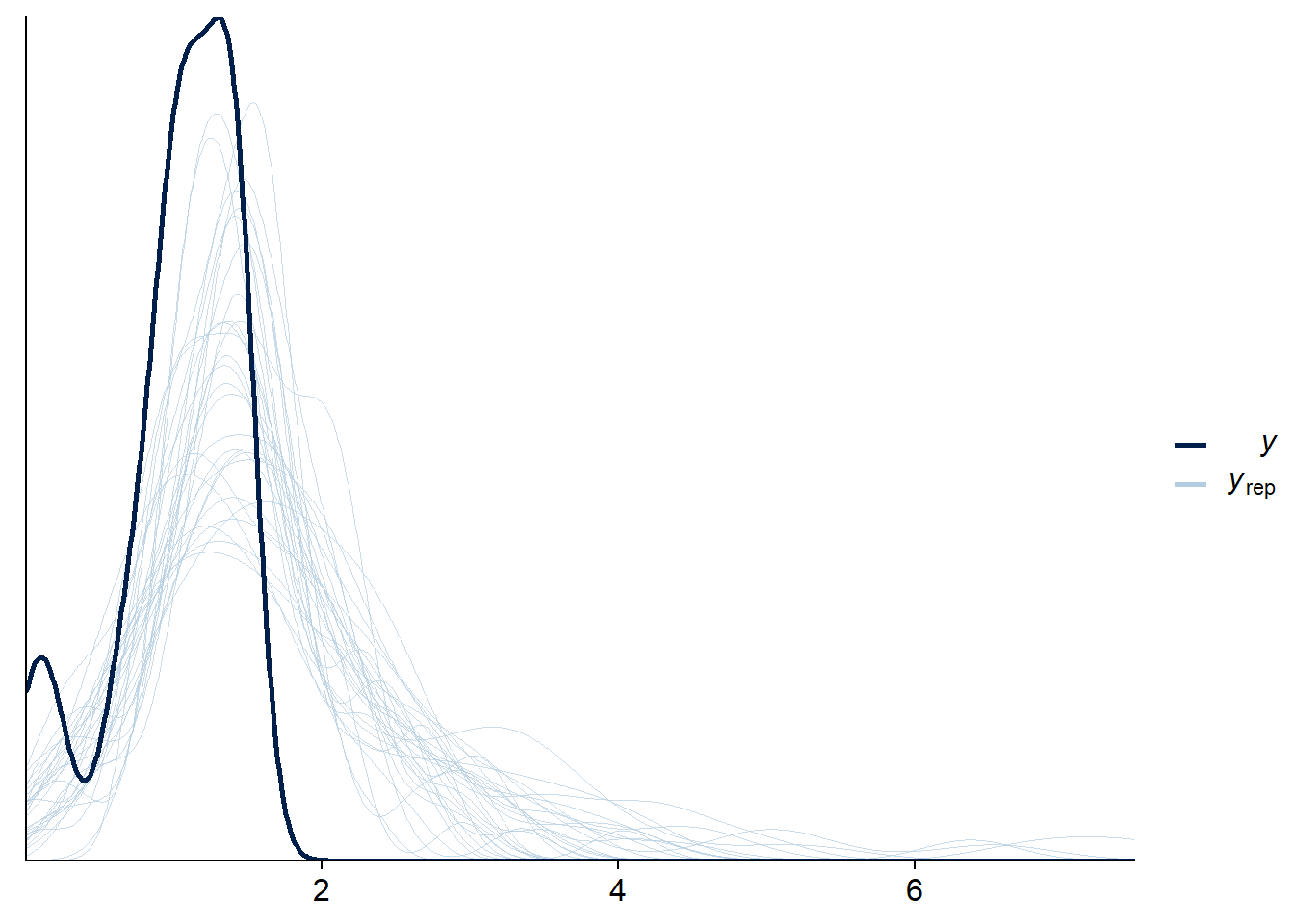But here we’ll also have to face possibly the biggest problem with brms: that it becomes very easy to specify a model that is too complex to be well informed by the data we have or to even build a completely broken model that no amount of data will save. The data and a few settings for the “crazy” models shown above have actually had to be tweaked for them to work well for this post. So enjoy with moderation :-).

Again, if you want the complete code, packaged in a single file you can just load into your project, go to https://github.com/martinmodrak/blog/blob/master/content/post/RTmixture.R

If you encounter problems running the models that you can’t resolve yourself, be sure to ask questions on Stan Discourse and tag me (@martinmodrak) in the question!

## Original computing environment

sessionInfo()
## R version 4.1.0 (2021-05-18)
## Platform: x86_64-w64-mingw32/x64 (64-bit)
## Running under: Windows 10 x64 (build 18363)
##
## Matrix products: default
##
## locale:
##  LC_COLLATE=English_United States.1252
##  LC_CTYPE=English_United States.1252
##  LC_MONETARY=English_United States.1252
##  LC_NUMERIC=C
##  LC_TIME=English_United States.1252
##
## attached base packages:
##  stats     graphics  grDevices utils     datasets  methods   base
##
## other attached packages:
##   bayesplot_1.8.1     patchwork_1.1.1     knitr_1.33
##   forcats_0.5.1       stringr_1.4.0       dplyr_1.0.7
##  tibble_3.1.3        ggplot2_3.3.5       tidyverse_1.3.1
##  brms_2.16.2         Rcpp_1.0.7          cmdstanr_0.4.0.9000
##
## loaded via a namespace (and not attached):
##    igraph_1.2.6         splines_4.1.0        crosstalk_1.1.1
##    rstantools_2.1.1     inline_0.3.19        digest_0.6.27
##   htmltools_0.5.1.1    rsconnect_0.8.24     fansi_0.5.0
##   magrittr_2.0.1       checkmate_2.0.0      tzdb_0.1.2
##   modelr_0.1.8         RcppParallel_5.1.4   matrixStats_0.61.0
##   xts_0.12.1           prettyunits_1.1.1    colorspace_2.0-2
##   rvest_1.0.1          haven_2.4.3          xfun_0.25
##   callr_3.7.0          crayon_1.4.1         jsonlite_1.7.2
##   lme4_1.1-27.1        zoo_1.8-9            glue_1.4.2
##   gtable_0.3.0         V8_3.4.2             distributional_0.2.2
##   pkgbuild_1.2.0       rstan_2.26.3         abind_1.4-5
##   scales_1.1.1         mvtnorm_1.1-2        DBI_1.1.1
##   miniUI_0.1.1.1       xtable_1.8-4         stats4_4.1.0
##   httr_1.4.2           threejs_0.3.3        posterior_1.0.1
##   ellipsis_0.3.2       pkgconfig_2.0.3      loo_2.4.1
##   farver_2.1.0         sass_0.4.0           dbplyr_2.1.1
##   utf8_1.2.2           labeling_0.4.2       tidyselect_1.1.1
##   rlang_0.4.11         reshape2_1.4.4       later_1.2.0
##   munsell_0.5.0        cellranger_1.1.0     tools_4.1.0
##   cli_3.0.1            generics_0.1.0       broom_0.7.9
##   ggridges_0.5.3       evaluate_0.14        fastmap_1.1.0
##   yaml_2.2.1           processx_3.5.2       fs_1.5.0
##   nlme_3.1-152         mime_0.11            projpred_2.0.2
##   xml2_1.3.2           compiler_4.1.0       shinythemes_1.2.0
##   rstudioapi_0.13      curl_4.3.2           gamm4_0.2-6
##   reprex_2.0.1         bslib_0.2.5.1        stringi_1.7.3
##   highr_0.9            ps_1.6.0             blogdown_1.5
##   Brobdingnag_1.2-6    lattice_0.20-44      Matrix_1.3-3
##   nloptr_1.2.2.2       markdown_1.1         shinyjs_2.0.0
##   tensorA_0.36.2       vctrs_0.3.8          pillar_1.6.2
##   lifecycle_1.0.0      jquerylib_0.1.4      bridgesampling_1.1-2
##  cowplot_1.1.1        httpuv_1.6.1         R6_2.5.0
##  bookdown_0.22        promises_1.2.0.1     gridExtra_2.3
##  codetools_0.2-18     boot_1.3-28          colourpicker_1.1.0
##  MASS_7.3-54          gtools_3.9.2         assertthat_0.2.1
##  withr_2.4.2          shinystan_2.5.0      mgcv_1.8-35
##  parallel_4.1.0       hms_1.1.0            grid_4.1.0
##  coda_0.19-4          minqa_1.2.4          rmarkdown_2.10
##  shiny_1.6.0          lubridate_1.7.10     base64enc_0.1-3
##  dygraphs_1.1.1.6